Distance Formula – How to UseDistance Formula – How to Use

Learn the distance formula and how to use it to find the distance between two points in this free math video tutorial by Mario’s Math Tutoring.

0:12 Distance Formula
0:56 Example 1 Finding Distance from (4,6),(7,10)
2:08 Example 2 Finding Distance from (-5,-2),(-9,-4)

Related Videos:

Finding the Midpoint

Learn Algebra 1 Lesson by Lesson in my “Learn Algebra 1” video course for sale here:
https://mariosmathtutoring.teachable.com/p/learn-algebra-1-video-course

Looking to raise your math score on the ACT and new SAT?
Check out my Huge ACT Math Video Course and my Huge SAT Math Video Course for sale at
http://mariosmathtutoring.teachable.com

http://www.mariosmathtutoring.com

* Organized List of My Video Lessons to Help You Raise Your Scores & Pass Your Class. Videos Arranged by Math Subject as well as by Chapter/Topic. (Bookmark the Link Below)
http://www.mariosmathtutoring.com/free-math-videos.html

algebra tutors,act math distance formula,mario’s math tutoring,distance between 2 points,Distance Formula,how to use the distance formula,what is the distance formula?,distance,pythagorean theorem,act math,act math concepts,algebra,math,finding distance,free math videos,video,free,tutorial,geometry

algebratutors.org

13 Responses to “Distance Formula – How to Use”

1.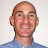Mario's Math Tutoring says:

https://mariosmathtutoring.teachable.com/p/learn-algebra-1-video-course
Check out my Learn Algebra 1 Video Course for sale. I teach you chapter by chapter and section by section. There are 87 video lessons and over 11 hours of video. For less than the price of a private tutoring session you have lifetime access to the course and can work through it at your own pace. Check out the 13 free lessons (1 from each chapter) at the link above.
– Mario

2.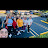ping IQ says:

Sorry, but my teacher got nothing on you when it comes to explaining

3.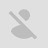Nolan Canavan says:

thank you mario

4.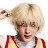penelope. says:

BRUH TYSM

5.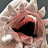U_can’t_see_me_im_too_small says:

You saved me from getting a whooping from failing the assignment

6.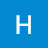SPARKLY PANDACORN says:

God bless you! You explained it perfectly thank you so much

7.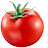Ben Z says:

Pov your math teacher sent you to watch this

8.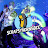Scrapsyhornsbee says:

Ma man’s math is on point… doesn’t even need to whip out the calculator

9.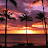TVD Fan says:

Thank you so much my teacher teaches us something new everyday and moves way to fast.pls continue to upload vids

10.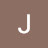LBM Meezy says:

Moving way to fast

11.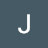Jagu Dada says:

tel lagan di lod nahi

12.Jagu Dada says:

or phir ganjy

13.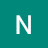Nasir Sabaghi says:

nice